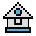Home

# SGN Function

#### Purpose:

To return the sign of x.

#### Syntax:

`SGN(x)`

x is any numeric expression.

If x is positive, SGN(x) returns 1.

If x is 0, SGN(x) returns 0.

If x is negative, SGN(x) returns -1.

This statement is similar to, but not the same as SIN(x), which returns a trigonometric function in radians, rather than in ones and zeros.

#### Examples:

```10 INPUT "Enter value", x
20 ON SGN(X)+2 GOTO 100, 200, 300```

GW-BASIC branches to 100 if X is negative, 200 if X is 0, and 300 if X is positive.